# Basic Statistics for Trading Strategies (Part 2) - Distribution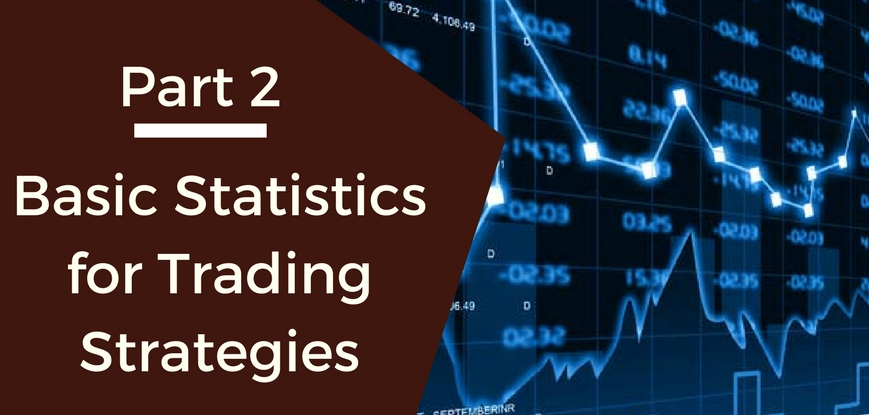This series of posts is to get our readers to start using statistics and data analysis while trading. In our first post, we discussed summary statistics such as mean, standard deviation, volatility & Bollinger bands.

In this post, we will try to understand distributions. This post also tries to answer the basic question: “why is statistics necessary for strategy building?” For this post, we will use R which has in-built statistical functions for easy analysis. You can download and install R-studio on your system to work along.

We will continue working with the dataset used in the previous blog post: MARUTI SUZUKI India Limited- Daily data from Jan 01, 2013 to Dec 31, 2013.

### Histograms

If we plot the closing prices as histograms or frequency distribution this is what we see. It basically plots the number of times the prices were between different ranges (1200-1300, 1300-1400, so on).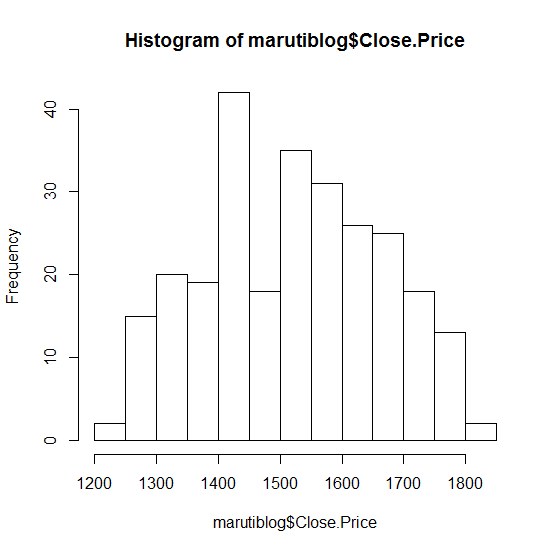R code:

```marutiblog <- read.csv(file = "Maruti_data.csv", header = TRUE)

hist(marutiblog\$Close.Price)```

#### What does this chart tell you?

It tells us that the closing prices of Maruti stock in the year 2013 lied between 1200 and 1800, with almost 50% of the times between 1400 and 1600. The shape of the distribution is almost a normal or bell curve with the mean at 1500.

#### A normal distribution

When the distribution of your data meets certain requirements, such as symmetry around the mean and bell-shaped curve, we say your data is normally distributed.

Statistically speaking, if X is Normally distributed with mean µ and standard deviation σ, we write X∼N(µ, σ2), µ and σ are the parameters of the distribution.

#### Why is it useful to know the distribution function of your dataset?

If you know that your data sample is, say, normally distributed, you can make ‘predictions’ about your population with certain ‘confidence’.

For example, say, your data sample X represents marks obtained out of 100 in an entrance test for a sample of students. The data is normally distributed such as X∼N(50, 102). When plotted this data would look as below: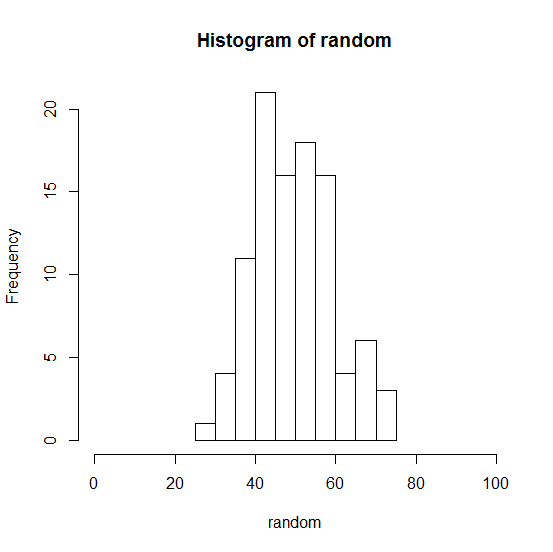R codes:

```random <- rnorm(100, mean = 50, sd = 10)

hist <- (random, xlim = c(0, 100), plot = TRUE)```

If you increase the number of observations in your sample data set from 100 to 1000, this is what happens: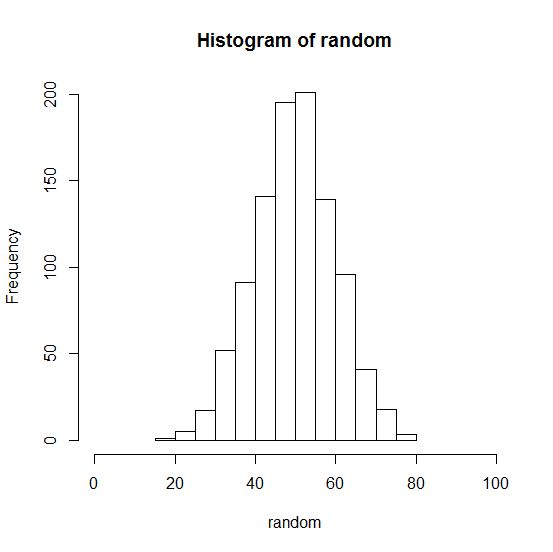It looks more bell-shaped!

Now that we know, X is normally distributed data with mean at 50 and standard deviation of 10, we can predict the marks of the entire student population or future students (from the same population) with a certain confidence. With almost 99.7% confidence, we can say that students would not get less than 20 or greater than 80 marks. With 95% confidence, we can say that students would get marks between 30 and 70 points.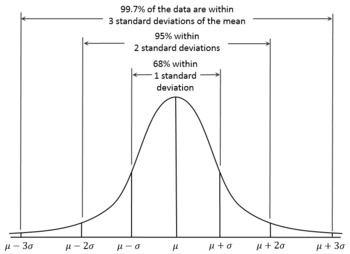Image source: http://en.wikipedia.org/wiki/Normal_distribution

Statistically speaking, distribution functions give us the probability of expecting the value of a given observation between two points. Hence, using distribution functions, also called probability density functions, we can ‘predict’ with certain ‘confidence’.

#### Are closing prices normally distributed?

A simple test called Normal Quantile-Quantile (qq) plots helps us find out if a set of observations is approximately normally distributed. A normal qq plot will result in an approximately straight line. For the closing prices, the qqline is almost a straight line: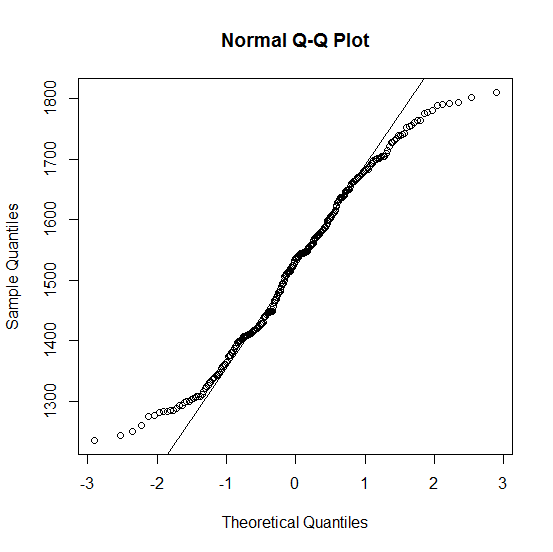This is not a perfect fit over a data and we can loosely say that the prices are normally distributed.

R code:

```> close.price <- marutiblog\$Close.Price

> qqnorm(close.price)

qqline(close.price)```

### Log returns Vs Simple returns

Now that we are introduced to distribution functions, let us think about log returns calculated and used in financial modeling. Log returns or continuously compounded returns are often used over simple returns for financial calculations. One main reason for doing so is the ease of multiplicative calculations of log values. We know, log (a/b) = log a – log b. To find cumulative returns over a period of time, one can simply add the daily log returns.

Another reason for choosing log returns over simple returns is that when we assume prices to follow a log-normal distribution, then log returns are normally distributed. This assumption is useful for working with classic statistics which rely on normality conditions.

Plotting log returns for the closing prices of the same dataset, we see the following chart. This shows that log returns for our data only loosely fit the normality conditions.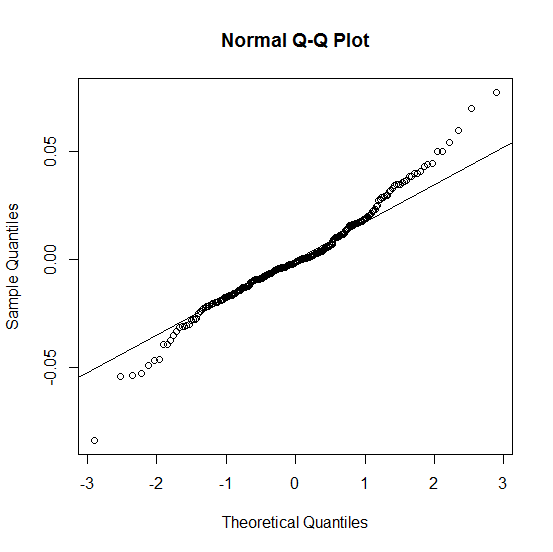To sum it up, statistics are used in every step of technical analysis and it is the core of the quantitative analysis. These analyses constitute the core part of any strategy building process.